Courses

# VITEEE Physics Test - 4

## 40 Questions MCQ Test VITEEE: Subject Wise and Full Length MOCK Tests | VITEEE Physics Test - 4

Description
This mock test of VITEEE Physics Test - 4 for JEE helps you for every JEE entrance exam. This contains 40 Multiple Choice Questions for JEE VITEEE Physics Test - 4 (mcq) to study with solutions a complete question bank. The solved questions answers in this VITEEE Physics Test - 4 quiz give you a good mix of easy questions and tough questions. JEE students definitely take this VITEEE Physics Test - 4 exercise for a better result in the exam. You can find other VITEEE Physics Test - 4 extra questions, long questions & short questions for JEE on EduRev as well by searching above.
QUESTION: 1

Solution:
QUESTION: 2

Solution:
QUESTION: 3

### Fraunhofer spectrum is a

Solution:
QUESTION: 4

The given graph shows the variation of charge q versus potential difference for two capacitors C1 and C2. The two capacitors have same plate separation, but the plate area of C2 is double that of C1.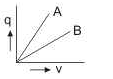Solution:As q = CV so, C = q/V and graph A has a larger slope than B,
So the graph A represents a capacitor of larger capacitance
Also, C = ℇ0A/d hence: C α A
As the plate area of C2 is double of that of C1,
So C2 has a larger capacitance
Hence the line A of the graph corresponds to C2

QUESTION: 5

A cell of e.m.f. E is connected with an external resistance R, then p.d. across cell is V. The internal resistance of cell will be

Solution: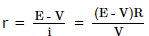QUESTION: 6

The resistance of a cell does not depend upon

Solution:
QUESTION: 7

If sky wave with a frequency of 50 MHz is incident on D-region at an angle of 30o, then angle of refraction is

Solution:
QUESTION: 8

The resistance of a wire of iron is 10 ohms and temp. coefficient of resistivity is 5x10-3∕℃. At 20oC it carries 30 milliamperes of current. Keeping constant potential difference between its ends, the temperature of the wire is raised to 120oC. The current in milliamperes that flows in the wire is

Solution:
QUESTION: 9

A wire of resistance 1 Ω is stretched to double its length. The resistance will become

Solution:
QUESTION: 10

Choke coil works on the principle of

Solution:
QUESTION: 11

If the flux of magnetic induction through a coil of resistance R and having N turns changes from φ1 to φ2 , then the magnitude of the charge that passes through this coil is

Solution:
QUESTION: 12

The wing span of an aeroplane is 36 m. If the plane is flying at 400 km/h, the e.m.f. induced between the wings tips is (assume V=4X10)

Solution:
QUESTION: 13

The electromagnetic waves are produced by

Solution:
QUESTION: 14

A cylinder of radius R and length L is placed in a uniform electric field E parallel to the cylinder axis, the total electric flux for the surface of the cylinder is

Solution:

Total charge enclosed is zero

QUESTION: 15

Two charges -10 C and + 10 C are placed 10cm apart. Potential at the centre of the line joining two charges is

Solution:
QUESTION: 16

A spherical shell of radius a with charge Q is expanded to radius b. The work done by the electric force in the process is

Solution:
QUESTION: 17

What will be the ratio of de Broglie wavelengths of proton and α-particle of same energy

Solution: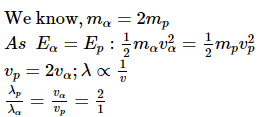QUESTION: 18

A body of capacity 4 μ F is charged to 80V and another body of capacity 6 μ F is charged to 30V. When they are connected the energy lost by 4 μ F is

Solution: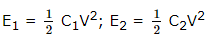QUESTION: 19

A wire of radius r has resistance R. If it is stretched to a radius of 3r/4, its resistance becomes

Solution:

Let L1 be the length and A1 the area of crosssection of the original wire and L2, A2 be their values of the stretched wire
Since volume of the wire remains unchanged, we have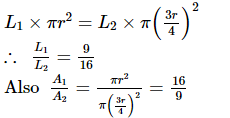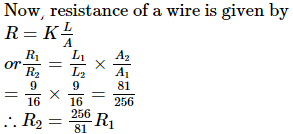QUESTION: 20

An electric dipole of dipole moment p is placed in uniform electric field of strength E. If θ is the angle between p and E, then potential energy of the dipole becomes maximum, when θ is

Solution:
QUESTION: 21

A capacitor of 10 μF charged up to 250 volts is connected in parallel with another capacitor of 5 μF charged up to 100 volts. The common potential is

Solution:
QUESTION: 22

Magnetic effect of current was discovered by

Solution:
QUESTION: 23

Substances which have identical chemical properties but differ in atomic weight are called

Solution:
QUESTION: 24

Number of neutrons in C12 and C14 are

Solution:
QUESTION: 25

In a nuclear reactor heavy water is used as a

Solution:
QUESTION: 26

The work function for tungsten and sodium are 4.5 eV and 2.3 eV respectively. If the threshold wavelength λ for sodium is 5460Å , the value of λ for tungsten is

Solution:
QUESTION: 27

The work function of a substance is 4.0 eV. The longest wavelength of light that can cause photoelectron emission from this substance is approximately

Solution:
QUESTION: 28
When Arsenic is added as an impurity to Silicon the resulting material is ---- semiconductor.
Solution:
QUESTION: 29
A ray of light strikes a glass plate at an angle θ. If angle between the reflected and refracted rays is a right angle, then refractive index of the glass plate is given by
Solution:
QUESTION: 30
The potential barrier, in the depletion layer, is due to
Solution:
QUESTION: 31

When plate voltage in diode valve is increased from 100 volt to 150 volt then plate current increases from 7.5 mA to 12 mA. The dynamic plate resistance will be

Solution:
QUESTION: 32

A prism of refractive index ' μ ' and angle 'A' is placed in the minimum deviation position. If the angle of minimum deviation is 'A', then the value of 'A' in terms of ' μ ' is

Solution:
QUESTION: 33

In a good conductor the energy gap between the conduction band and the valence band is

Solution:
QUESTION: 34
When arsenic is added as an impurity to silicon, the resulting material is
Solution:
QUESTION: 35

To make P-type semiconductor, the impurity to be mixed with pure germanium will be

Solution:
QUESTION: 36

In forward bias the width of potential barrier in a p-n junction diode

Solution:
QUESTION: 37

In Young's experiment of double slit, the intensity of central bright band is ---- times the individual intensities of the interfering waves

Solution:
QUESTION: 38

Two waves of intensities I and 4 I superimpose, then maximum and minimum intensities are

Solution: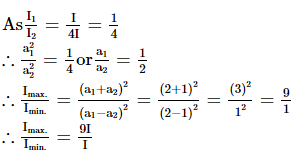QUESTION: 39

The phase difference between any two points on a wavefront is

Solution:
QUESTION: 40

If a star is moving towards the earth, then the spectrum lines are shifted towards

Solution: